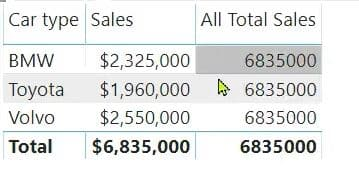Select Page

# VIDEO: FILTER and ALL Inside CALCULATE Function

by | Jul 28, 2020 | Video

There are many different ways to use the CALCULATE function in DAX. Depending on a task, you might want to put other DAX functions, such as FILTER and ALL, inside CALCULATE.

Two common functions that you put inside CALCULATE are the ALL and FILTER functions.

Watch our simple examples in a video to understand how to use ALL and FILTER.

### ALL function

The ALL function returns all the rows in a table or all the values in columns, ignoring all filters. This is helpful when clearing filters and creating calculations on all the rows in a table. This is why the ALL function is not used by itself, but serves as an intermediate function that can be used to change the set of results. After that, other calculations can be performed.

In our video, we used the following example:

All Sales = CALCULATE ( SUM (Sales[Sales Amount]), ALL (Sales) )After calculating these two measures, we can divide one by another to find out the % ratio of sales.

### FILTER function

The FILTER is a table function, which returns a table of values. Often, the function is used as a filter parameter inside the CALCULATE function.

In our example, let’s say we need to find out the number of days when the car sales were over \$200,000. In Excel, we would use the COUNTIF function. However, DAX doesn’t have COUNTIF and that’s why we’ll use FILTER:

Number of days with sales over 200k = CALCULATE (COUNT (Sales[TransactionID]),

FILTER (Sales, [Sales Amount] > 200000))

FILTER can filter rows from a table by any expression valid in the row context.

If there is a need to filter between two values, for example, between \$90 and \$100, you can use the AND (&&) operator. See the example below:

Sales between \$90 and \$100 = CALCULATE ( COUNT (Sales [TransactionID]),

FILTER ( Sales, Sales [Amount] > 95 && Sales [Amount] < 100))

In sum, the CALCULATE function in DAX provides you with some very interesting ways to calculate various measures. Depending on your needs, you can use functions, such as ALL and FILTER, inside CALCULATE.

## Tags:

DAX | Power BI | video